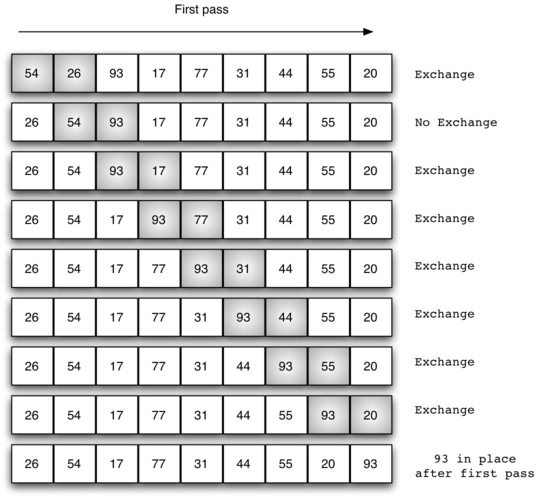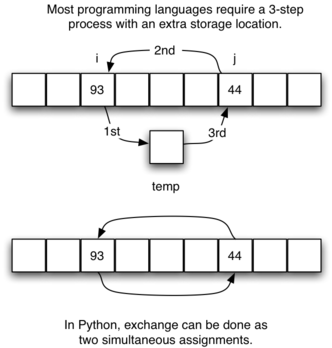# 7.3. The Bubble Sort¶

The bubble sort makes multiple passes through an array. It compares adjacent items and exchanges those that are out of order. Each pass through the array places the next largest value in its proper place. In essence, each item “bubbles” up to the location where it belongs.

Figure 1 shows the first pass of a bubble sort. The shaded items are being compared to see if they are out of order. If there are n items in the array, then there are $$n-1$$ pairs of items that need to be compared on the first pass. It is important to note that once the largest value in the array is part of a pair, it will continually be moved along until the pass is complete.Figure 1: bubbleSort: The First Pass

At the start of the second pass, the largest value is now in place. There are $$n-1$$ items left to sort, meaning that there will be $$n-2$$ pairs. Since each pass places the next largest value in place, the total number of passes necessary will be $$n-1$$. After completing the $$n-1$$ passes, the smallest item must be in the correct position with no further processing required. ActiveCode 1 shows the complete bubbleSort function. It takes the array as a parameter, and modifies it by swapping items as necessary.

Typically, swapping two elements in an array requires a temporary storage location (an additional memory location). A code fragment such as

temp = alist[i];
alist[i] = alist[j];
alist[j] = temp;


will exchange the ith and jth items in the array. Without the temporary storage, one of the values would be overwritten.

Lines 5-7 in ActiveCode 1 perform the exchange of the $$i$$ and $$(i+1)th$$ items using the three–step procedure described earlier. Note that we could also have used the simultaneous assignment to swap the items.Figure 2: Exchanging Two Values in Python

The following activecode example shows the complete bubbleSort function working on the array shown above.

The following animation shows bubbleSort in action. The sort compares two items at a time. Once it finds two out of place blocks it will find the correct place for the smaller block and then resets for another pass through.

This visualization allows you to step through the algorithm. Red bars represent the elements being looked at.

To analyze the bubble sort, we should note that regardless of how the items are arranged in the initial array, $$n-1$$ passes will be made to sort an array of size n. Table 1 shows the number of comparisons for each pass. The total number of comparisons is the sum of the first $$n-1$$ integers. Recall that the sum of the first n integers is $$\frac{1}{2}n^{2} + \frac{1}{2}n$$. The sum of the first $$n-1$$ integers is $$\frac{1}{2}n^{2} + \frac{1}{2}n - n$$, which is $$\frac{1}{2}n^{2} - \frac{1}{2}n$$. This is still $$O(n^{2})$$ comparisons. In the best case, if the array is already ordered, no exchanges will be made. However, in the worst case, every comparison will cause an exchange. On average, we exchange half of the time.

Table 1: Comparisons for Each Pass of Bubble Sort

Pass

Comparisons

1

$$n-1$$

2

$$n-2$$

3

$$n-3$$

$$n-1$$

$$1$$

A bubble sort is often considered the most inefficient sorting method since it must exchange items before the final location is known. These “wasted” exchange operations are very costly. However, because the bubble sort makes passes through the entire unsorted portion of the array, it has the capability to do something most sorting algorithms cannot. In particular, if during a pass there are no exchanges, then we know that the array must be sorted. A bubble sort can be modified to stop early if it finds that the array has become sorted. This means that for arrays that require just a few passes, a bubble sort may have an advantage in that it will recognize the sorted array and stop. ActiveCode 2 shows this modification, which is often referred to as the short bubble.

Self Check

Q-2: Suppose you have the following array of numbers to sort: [19, 1, 9, 7, 3, 10, 13, 15, 8, 12] which array represents the partially sorted list after three complete passes of bubble sort?

• [1, 9, 19, 7, 3, 10, 13, 15, 8, 12]
• This answer represents three swaps. A pass means that you continue swapping all the way to the end of the list.
• [1, 3, 7, 9, 10, 8, 12, 13, 15, 19]
• Very Good
• [1, 7, 3, 9, 10, 13, 8, 12, 15, 19]
• A bubble sort contines to swap numbers up to index position passnum. But remember that passnum starts at the length of the list - 1.
• [1, 9, 19, 7, 3, 10, 13, 15, 8, 12]
• You have been doing an insertion sort, not a bubble sort.